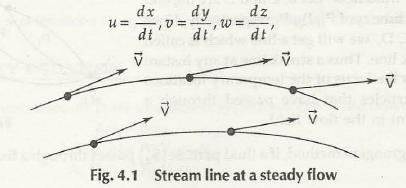Streamline is the flow of fluid where velocity remains constant at very fixed point. In other words, if the velocity vector is tangential to fluid flow curve, it is called streamline as shown in Fig. 4.1Let us consider u be the velocity in x-direction

v be the velocity in y-direction

w be the velocity in z-direction

It can be written as

u = [dx/dt]

v = [dy/dt]

And,

w = [dz/dt]

The equation of general stream line can be given by,

dx/u = dy/v = dz/w

For two rectangular coordinates, the above equation can be written as-

dx/u = dy/v

Note:

The above equation shows that slope of each stream line is equal to the ratio of respective velocity.

Links of Previous Main Topic:-

Links of Next Mechanical Engineering Topics:-

### Submit Your Assignment### Customer Reviews

My Homework Help
Rated 5.0 out of 5 based on 510 customer reviews at